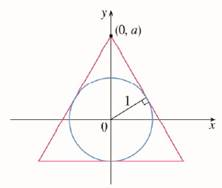Chapter 3.P, Problem 10P

Chapter
Section
Textbook Problem

# An isosceles triangle is circumscribed about the unit circle so that the equal sides meet at the point ( 0 , a ) on the y-axis (see the figure). Find the value of a that minimizes the lengths of the equal sides. (You may be surprised that the result does not give an equilateral triangle.).To determine

To find:

The value of a that minimizes the lengths of the equal sides.

Explanation

1) Concept:

Use implicit differentiation to unit circle to find the slope of AC- at point D then find the tangent line at D(b, c) to find the value of a that minimizes the lengths of the equal sides.

2) formula:

i. Power rule:ddxxn=nxn-1

ii. Quadratic formula: If ax2+bx+c=0 then x=-b±b2-4ac2a

iii. Quotient rule-ddxuv=v·ddxu-u·ddxvv2

3) Calculation:

Let AC- be tangent to the unit circle at point D.

To find the slope of AC- at point D.

Use implicit differentiation to unit circle.

x2+y2=1

Differentiate implicitly.

2x2-1+2y2-1y'=0

Simplify.

2x+2yy'=0

Subtract 2x and simplify.

2x+2yy'-2x=0-2x

2yy'=-2x

Divide by 2y on both sides.

2yy'2y =-2x2y

Simplify.

y'=-xy =-bc

Therefore, the tangent line at D(b, c) has equation.y=-bc x+a

At D, x=b, y=c

So put x=b, y=c in the tangent line equation. y=-bc x+a

c=-bc (b)+a

Simplify and add b2c  on both sides.

c+b2c =-b2c +a+b2c

Simplify and rearrange.

a=c2+b2c

Since c2+b2=1

a=1c

That is c=1a

Since c2+b2=1

Subtract c2 from both the sides.

c2+b2-c2=1-c2

Simplify.

b2=1-c2

Take square root of both the sides.

b2=1-c2

Simplify.

b=1-c2

Put c=1a  in the above equation.

=1-1a 2

Simplify.

b=a2-1a

At C, y=-1

So put y=-1 in the tangent line equation. y=-bc x+a

-1=-bc x+a

Add bc x+1 on both sides.

-1+bc x+1 =-bc x+a+bc x+1

Simplify

### Still sussing out bartleby?

Check out a sample textbook solution.

See a sample solution

#### The Solution to Your Study Problems

Bartleby provides explanations to thousands of textbook problems written by our experts, many with advanced degrees!

Get Started

#### Evaluate the integral. 3. esinxsecxdx

Single Variable Calculus: Early Transcendentals

#### ex(1e2x)3/2dx

Calculus: Early Transcendental Functions (MindTap Course List)

#### True or False: is a convergent series.

Study Guide for Stewart's Single Variable Calculus: Early Transcendentals, 8th